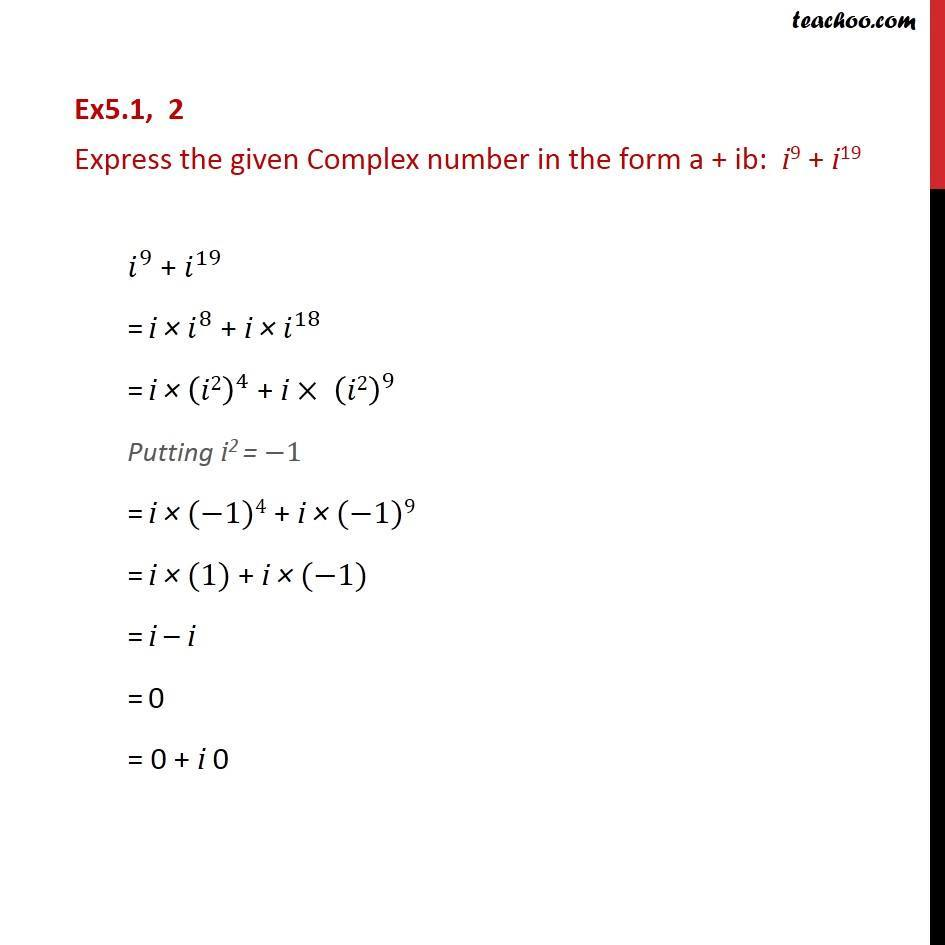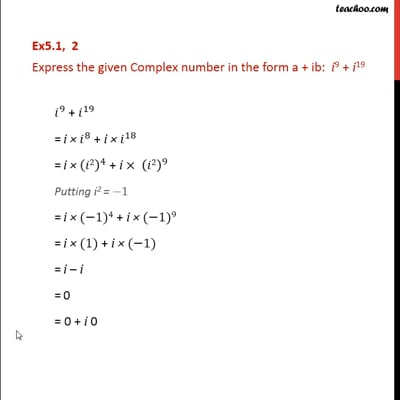Ex 5.1

Chapter 5 Class 11 Complex Numbers
Serial order wiseThis video is only available for Teachoo black users

Solve all your doubts with Teachoo Black (new monthly pack available now!)

### Transcript

Ex5.1, 2 Express the given Complex number in the form a + ib: i9 + i19 𝑖^9 + 𝑖^19 = i × 𝑖^8 + i × 𝑖^18 = i × (𝑖2)^4 + i × (𝑖2)^9 Putting i2 = −1 = i × (−1)4 + i × (−1)9 = i × (1) + i × (−1) = i – i = 0 = 0 + i 0